This article explores the statistical concept of science-wise false discovery rate (SWFDR). Some authors use SWFDR and its complement, positive predictive value, to argue that most (or, at least, many) published scientific results must be wrong unless most hypotheses are a priori true. I disagree. While SWFDR is valid statistically, the real cause of bad science is “Publish or Perish”.

## Introduction

Is science broken? A lot of people seem to think so, including some esteemed statisticians. One line of reasoning uses the concepts of false discovery rate and its complement, positive predictive value, to argue that most (or, at least, many) published scientific results must be wrong unless most hypotheses are a priori true.

The false discovery rate (FDR) is the probability that a significant p-value indicates a false positive, or equivalently, the proportion of significant p-values that correspond to results without a real effect. The complement, positive predictive value ($$PPV=1-FDR$$) is the probability that a significant p-value indicates a true positive, or equivalently, the proportion of significant p-values that correspond to results with real effects.

I became interested in this topic after reading Felix Schönbrodt’s blog post, “What’s the probability that a significant p-value indicates a true effect?” and playing with his ShinyApp. Schönbrodt’s post led me to David Colquhoun’s paper, “An investigation of the false discovery rate and the misinterpretation of p-values” and blog posts by Daniel Lakens, “How can p = 0.05 lead to wrong conclusions 30% of the time with a 5% Type 1 error rate?” and Will Gervais, “Power Consequences”.

The term science-wise false discovery rate (SWFDR) is from Leah Jager and Jeffrey Leek’s paper, “An estimate of the science-wise false discovery rate and application to the top medical literature”. Earlier work includes Sholom Wacholder et al’s 2004 paper “Assessing the Probability That a Positive Report is False: An Approach for Molecular Epidemiology Studies” and John Ioannidis’s 2005 paper, “Why most published research findings are false”.

## Scenario

Being a programmer and not a statistician, I decided to write some R code to explore this topic on simulated data.

The program simulates a large number of problem instances representing published results, some of which are true and some false. The instances are very simple: I generate two groups of random numbers and use the t-test to assess the difference between their means. One group (the control group or simply group0) comes from a standard normal distribution with $$mean=0$$. The other group (the treatment group or simply group1) is a little more involved:

• for true instances, I take numbers from a standard normal distribution with mean d ($$d>0$$);
• for false instances, I use the same distribution as group0.

The parameter d is the effect size, aka Cohen’s d.

I use the t-test to compare the means of the groups and produce a p-value assessing whether both groups come from the same distribution.

The program does this thousands of times (drawing different random numbers each time, of course), collects the resulting p-values, and computes the FDR. The program repeats the procedure for a range of assumptions to determine the conditions under which most positive results are wrong.

For true instances, we expect the difference in means to be approximately d and for false ones to be approximately 0, but due to the vagaries of random sampling, this may not be so. If the actual difference in means is far from the expected value, the t-test may get it wrong, declaring a false instance to be positive and a true one to be negative. The goal is to see how often we get the wrong answer across a range of assumptions.

## Nomenclature

To reduce confusion, I will be obsessively consistent in my terminology.

• An instance is a single run of the simulation procedure.
• The terms positive and negative refer to the results of the t-test. A positive instance is one for which the t-test reports a significant p-value; a negative instance is the opposite. Obviously the distinction between positive and negative depends on the chosen significance level.
• true and false refer to the correct answers. A true instance is one where the treatment group (group1) is drawn from a distribution with $$mean=d$$ ($$d>0$$). A false instance is the opposite: an instance where group1 is drawn from a distribution with $$mean=0$$.
• empirical refers to results calculated from the simulated data, as opposed to theoretical which means results calculated using standard formulas.

The simulation parameters are

parameter meaning default
prop.true fraction of cases where there is a real effect seq(.1,.9,by=.2)
m number of iterations 1e4
n sample size 16
d standardized effect size (aka Cohen’s d) c(.25,.50,.75,1,2)
pwr power. if set, the program adjusts d to achieve power NA
sig.level significance level for power calculations when pwr is set 0.05
pval.plot p-values for which we plot results c(.001,.01,.03,.05,.1)

## Results

The simulation procedure with default parameters produces four graphs similar to the ones below.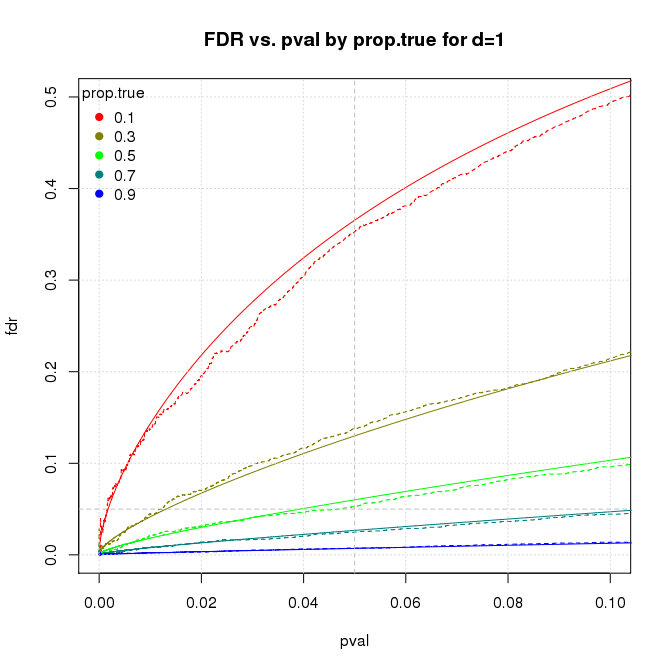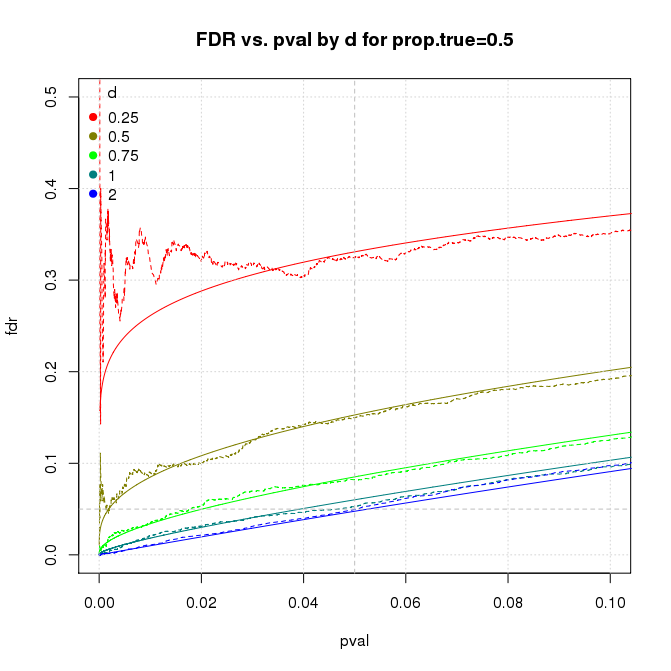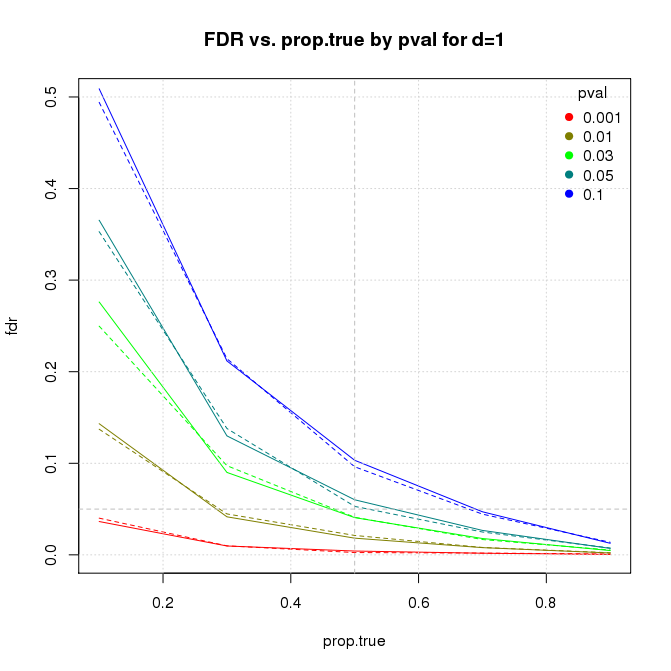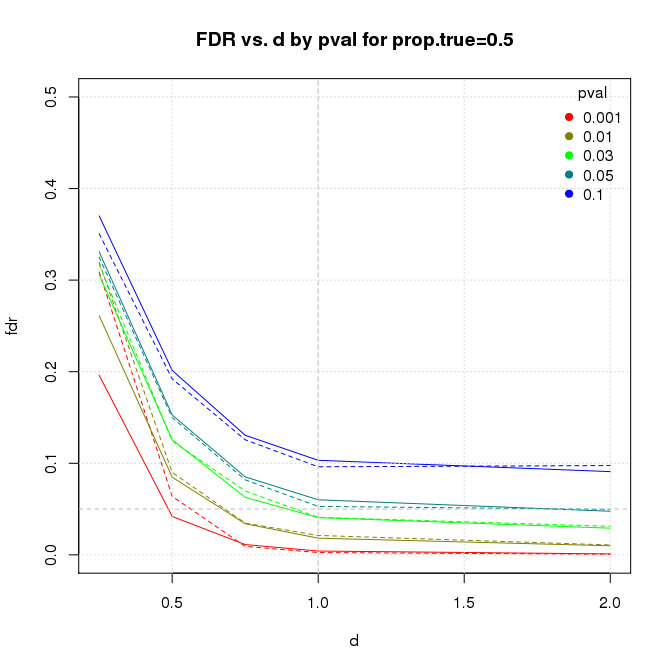In these graphs,

• solid lines show theoretical results; dashed lines are empirical results from the simulation
• fdr. false discovery rate
• pval. p-value cutoff for significance
• prop.true. proportion of true instances, i.e., ones that have a real effect
• d. standardized effect size, aka Cohen’s d

The first graph shows FDR vs. p-value across a range of prop.true values for a single effect size ($$d=1$$). Note the difference in x (p-value) and y (FDR) scales; the p-value scale is roughly an order of magnitude smaller than FDR. For this effect size, FDR behaves pretty well: for $$prop.true=0.5$$, FDR and p-value are pretty close; as prop.true gets smaller, FDR becomes larger than p-value; as prop.true gets larger, FDR shrinks below p-value. In other words, for this effect size, if most instances are true, p-values do a good job of separating the wheat from the chaff, but if most are false, p-values are less helpful. In the worse case plotted here, FDR is about 0.36 when $$pval=0.05$$.

The second graph shows FDR vs. p-value across a range of effect sizes for a single value of prop.true (0.5). Again note the difference in scales. Recall that FDR behaves pretty well for this value of prop.true when $$d=1$$. It’s still reasonable for $$d=0.75$$. But for smaller effect sizes, FDR again grows to be much larger than p-value. In the worse case plotted here, FDR is about 0.33 when $$pval=0.05$$.

We can also think of this in terms of power. As d gets smaller, so does power. The table below shows power for the default values of d. You’ll notice that power ranges from whopping good to anemic as we move from $$d=2$$ to $$d=0.25$$. For $$d=0.75$$, power is just over 50%; at this power, FDR is about .08 when $$pval=0.05$$. The table below shows FDR for all values of d under the conditions plotted here.

d 0.25 0.50 0.75 1.00 2.00
power 0.10 0.28 0.54 0.78 0.9998

The third graph shows FDR vs. prop.true across a range of p-values for a single effect size ($$d=1$$). In this graph, the x and y scales are about the same. For this effect size, FDR behaves pretty well until prop.true gets below 0.3. The inflection point at 0.3 is an artifact of the simulation; adding a few more prop.true values between 0.1 and 0.3 smooths out the curve (data not shown).

The final graph shows FDR vs. d across a range of p-values for a single value of prop.true (0.5). As d drops below 1, FDR grows rapidly as we’ve seen before. Reducing the p-value helps, as you would expect. But even with p-value=.001, FDR grows rapidly for $$d<0.5$$, reaching about 0.2 for $$d=0.25$$. This is because power is abysmal (.004) at this point causing us to miss most true instances. This illustrates the tradeoff between false positives and false negatives as we reduce the p-value: smaller p-values give fewer false positives but also fewer true positives.

Returning to the second graph above (FDR vs. p-value for a range of effect sizes and $$prop.true=0.5$$), we see that for small values of d and pval, the empirical results are noisy and don’t match the theoretical results very well. This is because there aren’t enough positives in this region. Increasing the number of simulations to $$10^6$$ fixes the problem as shown in the graph below.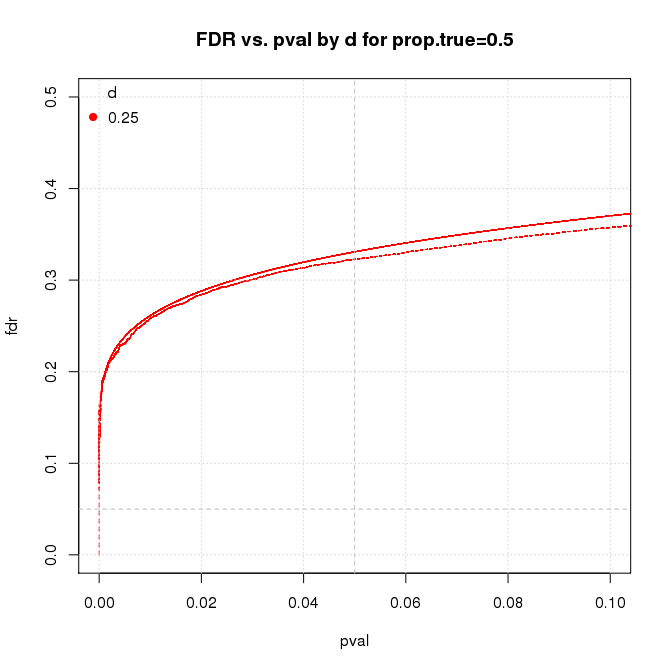The relationship between FDR and p-value is complicated. If prop.true is 50% or better and d is 1 or more, p-values do a good job at discriminating true from false instances. Under less optimistic conditions, p-values are not so good. Under the most pessimistic conditions here, FDR is about 1/3. Reducing the significance level improves FDR but at the cost of missing more true instances.

Let’s look at extreme cases of prop.true (0.25, 0.75) and power (0.2, 0.8) for pval=.05. The table below shows theoretical FDR for these cases.

high power low power
high prop.true 0.02 0.16
low prop.true 0.08 0.43

The best case is great (FDR=0.02), the worst case is horrible (0.43), and the in-between cases range from 0.08 to 0.16. The take-home is that if most hypotheses are wrong, you have to do good, well-powered studies to find the few correct results, but if most hypotheses are correct, you may be able to get by with sloppy science.

## Discussion

I started with the question, “Is science broken?” and segued to the more specific question of “Are most (or, at least many) published results wrong?” I then reported the claim that “Yes, most (or many) results must be wrong unless most hypotheses are a priori true”, because the science-wise false discovery rate (SWFDR) makes it so. Do the results here support the claim?

It depends on prop.true, so we’d better be clear about what it represents.

• David Colquhoun’s paper seems to suggest that it refers to early stage experiments. At one point the paper says, “[I]magine that we are testing a lot of candidate drugs, one at a time. It is sadly likely that many of them would not work, so let us imagine that 10% of them work and the rest are inactive.” In the on-line post-publication discussion, Dr. Colquhoun is even more explicit: “To postulate a prevalence greater than 0.5 is tantamount to saying that you were confident that your hypothesis was right before you did the experiment.”

• Felix Schönbrodt’s blog post has a similar statement: “It’s certainly not near 100% – in this case only trivial and obvious research questions would be investigated, which is obviously not the case.”

I disagree with this interpretation. Hypotheses exist at many stages of research from vague ideas flitting through students’ heads to more precise claims in published papers. Since we’re reasoning about the validity of published results, prop.true (and other parameters like d) must refer to hypotheses late in the research process, ones that are far enough along to be considered for publication. To understand prop.true, we need to understand how research shapes hypotheses.

What happens to the incorrect hypotheses that make it to the near-publication stage? I see three possibilities:

1. By (bad) luck, the study yielded a significant p-value, and the happy but hapless investigators proceed to publication.
2. The lab chief thinks the negative finding is correct and publishes the negative result or abandons the work. Sadly, this happens rarely, as we know too well.
3. The lab chief is unconvinced and sends the student back to the lab for more experiments or to the computer for more analyses.

How this unfolds depends on the skill and motivation of the people involved. If the student is good and driven by a quest for truth, and the lab chief provides enough support, case #3 will improve the initial hypothesis and yield one that’s true. If, on the other hand, the goal is simply to publish, this step is p-hacking and will produce a positive p-value whether or not the hypothesis is true.

Ignoring the rare case #2, all hypotheses that make it this far will eventually yield positive results and be published. This makes the work we’ve done simulating SWFDR totally irrelevant. The SWFDR we get will be close to whatever value we assume for the proportion of false hypotheses. In other words, $$FDR \approx 1-prop.true$$. Rather obvious, I think, and completely pointless.

I’ve seen plenty of bad science up close and personal and am thoroughly convinced that many published results in my field are rubbish. But I don’t buy the arguments based on SWFDR. The problem is p-hacking, both experimental and computational.

It’s really just another consequence of “Publish or Perish”. Those who can, publish good science; those who can’t, p-hack. No amount of statistical cleverness can change this basic dynamic. If you replace the much-maligned p-value by some other statistic s, p-hackers will become s-hackers, and the overall quality of science will remain unchanged. (See Paul Smaldino and Richard McElreath’s paper “The natural selection of bad science” for a much deeper treatment of this phenomenon.)

Good science drives the field forward; bad science is ephemeral. It’s aggravating to see so much dreck get published, but it’s even more aggravating to see good statisticians and data scientists agonizing over the ordure and spending so much effort trying to root out bad science. We will do more good by helping good scientists do good science than by trying to slow down the bad ones. Quoting the sage Oprah Winfrey (from BrainyQuote), “Be thankful for what you have; you’ll end up having more. If you concentrate on what you don’t have, you will never, ever have enough.”